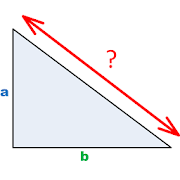## Diagonal helps you practice Grade HS Math - Lumos Educational App Store4.51
Price -fREE
\$0

#### DESCRIPTION:

Application is designed for carpenters, who wants to calculate balk length in a rectangular tirangle. Main functions: - calculates lengths of triangle sides - calculates area and perimeter - calculates angles and their sine, cosine, tangent and cotangent - tracks calculation history - allows to store calculations in Favourites tab

#### OVERVIEW:

Diagonal is a free educational mobile app By .It helps students in grades HS practice the following standards HSF.TF.C.8.

This page not only allows students and teachers download Diagonal but also find engaging Sample Questions, Videos, Pins, Worksheets, Books related to the following topics.

1. HSF.TF.C.8 : Prove the Pythagorean identity sin2(θ) + cos2(θ) = 1 and use it to find sin(θ), cos(θ), or tan(θ) given sin(θ), cos(θ), or tan(θ) and the quadrant of the angle..

HS

#### STANDARDS:

HSF.TF.C.8

Developer:

Software Version: 1.7

Category:

### RELATED APPSEdSearch WebSearch